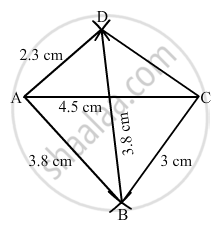Advertisement Remove all ads

# Construct a Quadrilateral Abcd in Which Ab = 3.8 Cm, Bc = 3.0 Cm, Ad = 2.3 Cm, Ac = 4.5 Cm and Bd = 3.8 Cm. - Mathematics

Answer in Brief

Construct a quadrilateral ABCD in which AB = 3.8 cm, BC = 3.0 cm, AD = 2.3 cm, AC = 4.5 cm and BD = 3.8 cm.

Advertisement Remove all ads

#### Solution

$\text { Steps of construction: }$

$\text { Step I: Draw AC = 6 cm }.$

$\text { Step II: With A as the centre and radius 3 . 8 cm, draw an arc } .$

$\text { Step III : With C as the centre and radius 3 . 0 cm, draw an arc to intersect the arc drawn in Step II at B }.$

$\text { Step IV: With B as the centre and radius 3 . 8 cm, draw an arc on the other side of AC }.$

$\text { Step V : With A as the centre and radius 2 . 3 cm, draw an arc to intersect the arc drawn in Step IV at D }.$

$\text { Step VI: Join BA, DA, BC and CD to obtained the required quadrilateral }.$Is there an error in this question or solution?
Advertisement Remove all ads

#### APPEARS IN

RD Sharma Class 8 Maths
Chapter 18 Practical Geometry (Constructions)
Exercise 18.2 | Q 1 | Page 6
Advertisement Remove all ads

#### Video TutorialsVIEW ALL 

Advertisement Remove all ads
Share
Notifications

View all notifications

Forgot password?
Course